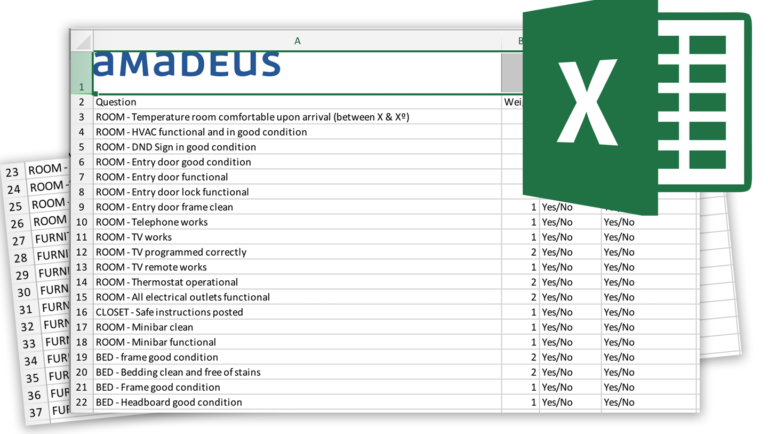Checklist

# RevPAR Worksheet## RevPAR Formula & Calculation [+Free RevPAR Calculator Excel]

Measure the overall health of your hotel with our easy-to-use worksheet.

### RevPAR Formula

RevPAR is one of the most important metrics used to determine revenue generating performance so that hotels can accurately price rooms. As a popular metric, RevPAR can also be used to help hotels measure their success against other brands and properties.

### RevPAR Calculation

To calculate RevPAR, simply multiply the average daily rate (ADR) by the occupancy rate.

So RevPar = [Occupancy Rate %] multiplied by [Average Daily Rate] (More information in the attached excel)

### Free RevPAR Calculator [Excel]

We know organizing data and calculating formulas is the last thing anyone wants to do, which is why we’ve created a free worksheet to streamline the process. As a bonus, this worksheet also includes calculations for ADR, ARR, and Occupancy Rate!